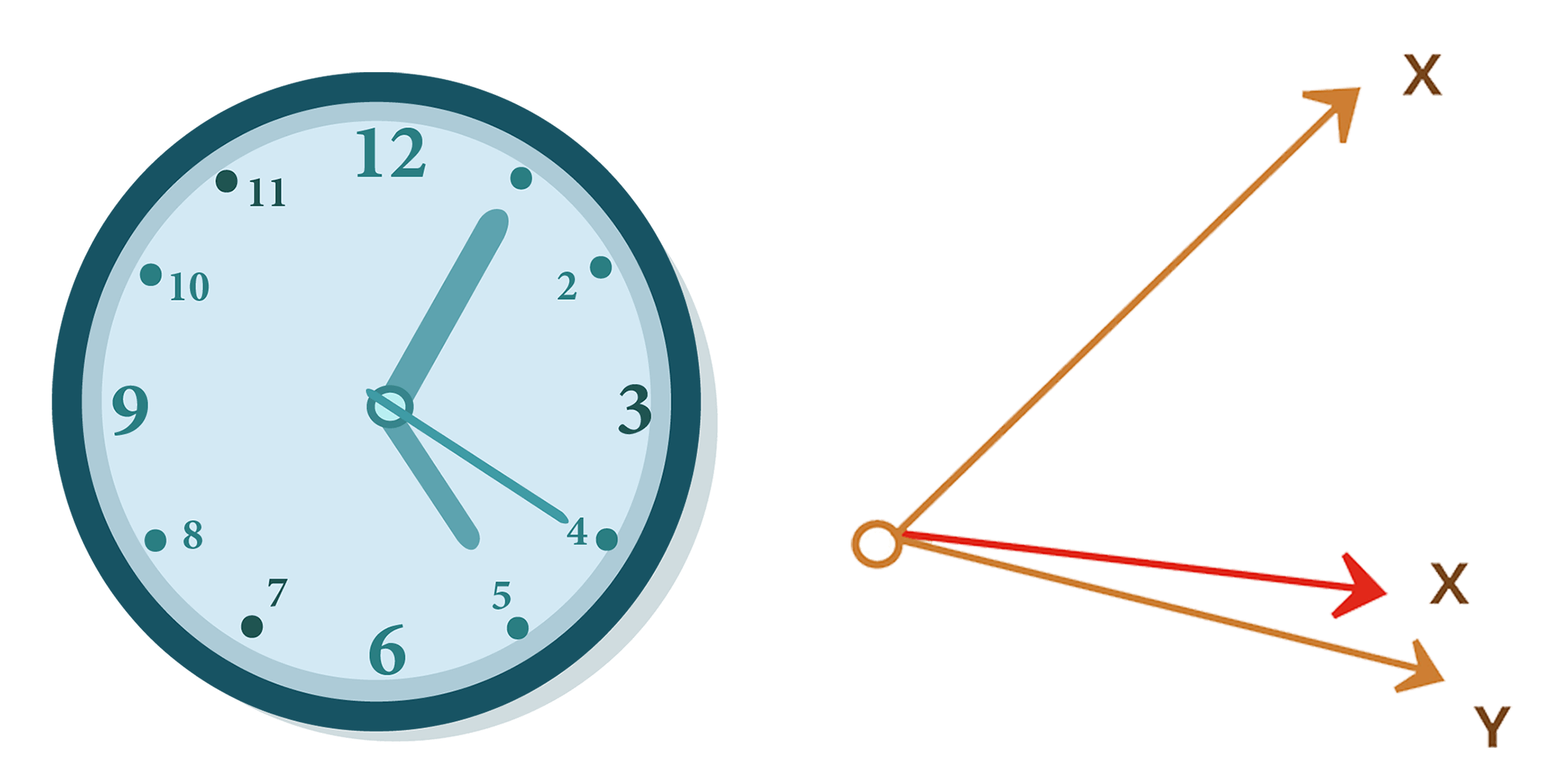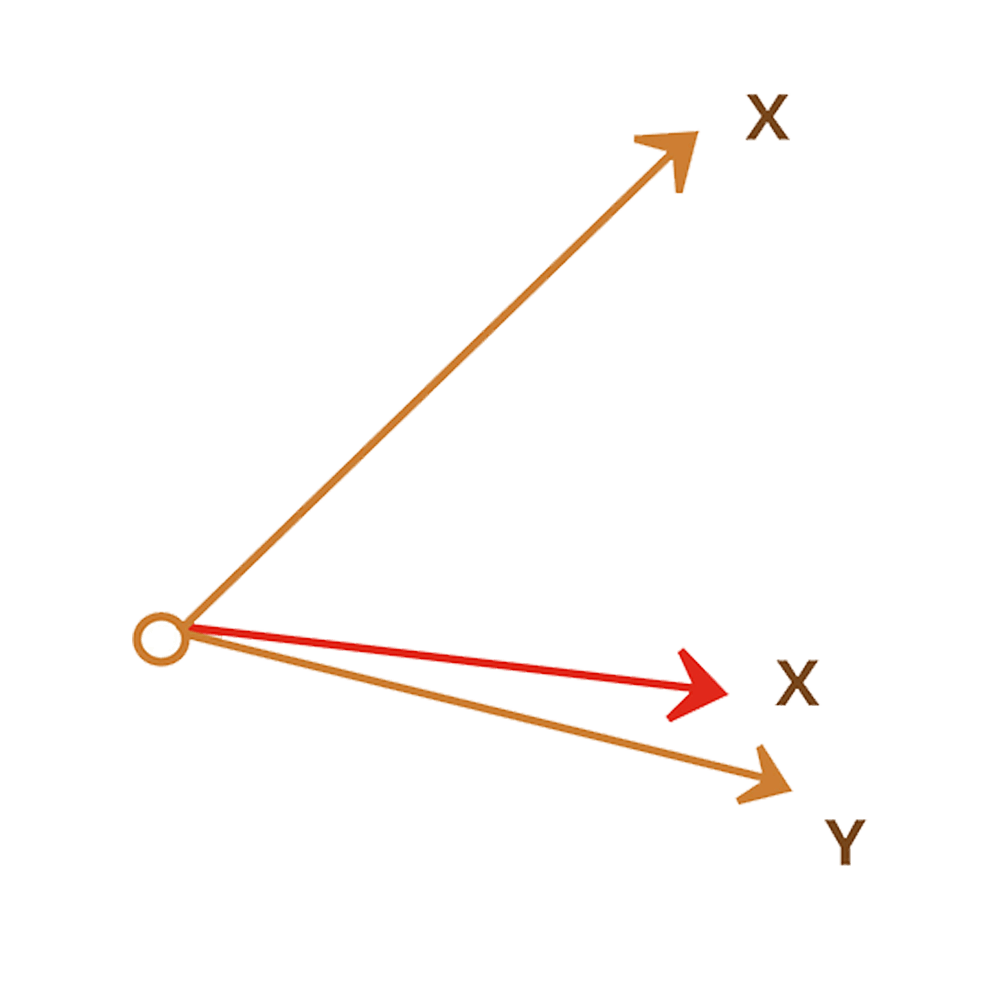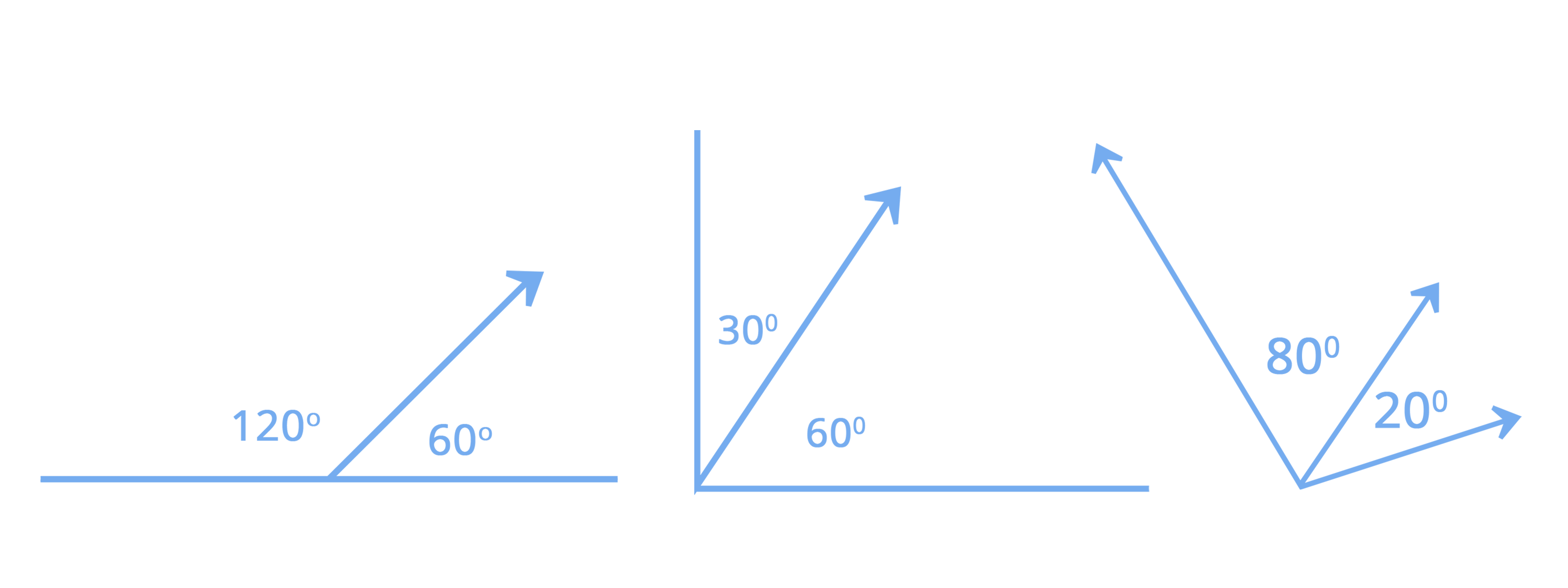• Call Now

1800-102-2727•

The angle created by the ray between its beginning and final positions is the measure of rotation of a ray when rotated about its terminus. The pair of angles is sometimes used in geometry. Complementary angles, neighboring angles, linear pairs of angles, opposing angles etc., are all examples of pairs of angles.We will go through the definitions of neighboring angles and vertical angles in-depth in this post.

When two angles have the same vertex and side, they are said to be neighbouring angles. This is because the vertex of an angle is formed by the ray's ends, which create the angle's side. On the other hand, when adjacent angles have the same vertex and side, they might be complementary or supplementary angles.

Take a look at a wall clock. The minute hand and the second hand of the clock make one angle, ∠XOZ, while the hour hand forms another angle, ∠ZOY, with the second hand. These two angles, ∠XOZ and ∠ZOY, are known as neighbouring angles since they are close to one other.The unusual arms are on each side of the common arms in ∠XOZ and ∠ZOY. They share a common vertex and a common arm. The next angles are those that are adjacent to one another.

The following are some of the significant properties of neighbouring angles:
Two angles are neighbouring if :

• They are in the same plane.
• They have the same vertex.
• They have the same arm.
• Angles do not intersect.
• There isn't a common interior point.
• When they share the same vertex, they might be complementary or supplementary angles.
• On both sides of the common arm, there should be a non-common arm.## Supplementary Angles Nearby

What is the total of the angles that are next to each other? Nearby angles will share the common side and vertex. If the total of two angles is 180 degrees, they are said to be supplementary angles. A linear pair is formed when two additional angles are close to each other.180 is the sum of two adjacent supplementary angles.

## Linear Pair

A linear pair is a group of neighbouring angles whose measurements add together to produce a straight angle. Thus, a linear pair's angles are complementary.

Consider the following diagram, in which a ray OP is depicted standing on the line segment AB:

At O, the angles ∠POA and ∠POB are created. ∠POA and ∠POB are neighbouring and supplementary angles, i.e. ∠POA + ∠POB = ∠AOB = 180°.

∠POB and ∠POA are next to each other, and when the total of neighbouring angles equals 180°, they create a linear pair of angles.

## Angles that are vertically opposed

Four angles are produced when two lines cross, as seen in the diagram below. ∠AOD and ∠BOC are vertically opposite each other, while ∠AOC and ∠DOB are also vertically opposed. Vertical angles or opposing angles are other names for these angles. As a result of the intersection of two lines, two pairs of vertically opposite angles are created, namely ∠DOB, ∠AOC, and ∠BOC, ∠AOD.The vertical angle theorem states that the vertically opposite angles of a pair of crossing lines are identical.

## Most Commonly Asked Questions- FAQs

Ques 1: What are Adjacent Angles, and How Do I Use Them?
Adjacent angles are defined as two angles that share the same vertex and side. Based on their total value, two neighbouring angles might be complementary or supplementary.

Ques 2: What do you mean by vertical angles?
When two lines cross, vertical angles are defined as the opposite angles (i.e. intersect). Vertically opposite angles is another name for it. Two vertical angles are always equal.

Ques 3: Is it possible for vertical angles to be adjacent?
No, vertical angles can't be next to each other. This is because adjacent angles are those that are adjacent to one other, whereas vertical angles are those that are opposite to one another.Talk to ourexpert
Resend OTP Timer =
By submitting up, I agree to receive all the Whatsapp communication on my registered number and Aakash terms and conditions and privacy policy